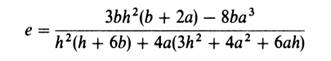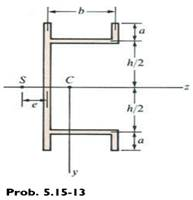### Create an Account

Home / Questions / Derive the following formula for the distance e from the centerline of the web to the shea...

# Derive the following formula for the distance e from the centerline of the web to the shear center S for the singly symmetric cross section of constant thickness shown in the figure Also check

Derive the following formula for the distance e from the centerline of the web to the shear center S for the singly symmetric cross section of constant thickness shown in the figure:Also, check the formula for the special case in which a = O (channel section).Jun 29 2020 View more View LessSubscribe To Get Solution